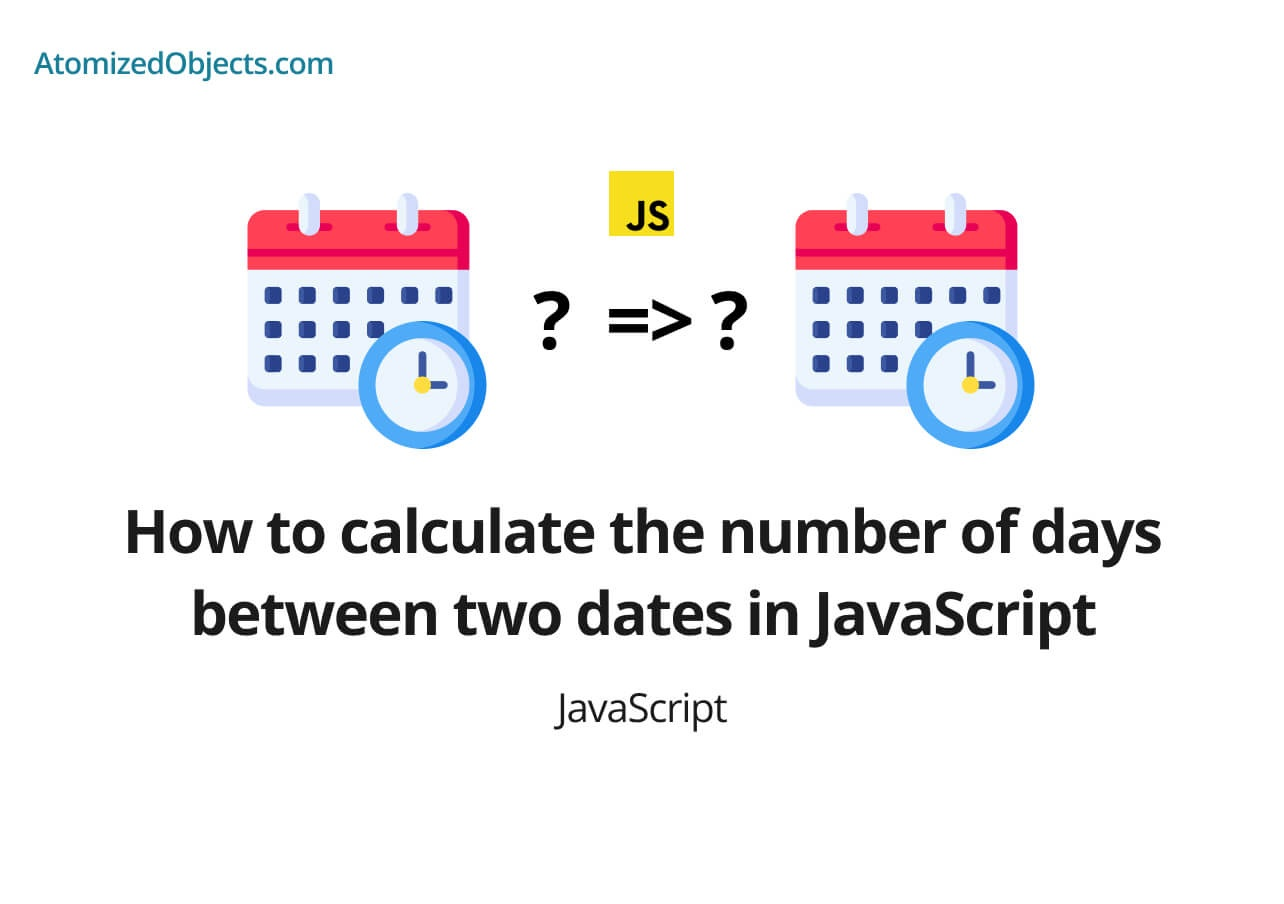Blog / Javascript

# How to calculate the number of days between two dates in JavaScript​​In this post find out how to to calculate the number of days between two dates in JavaScript​​ with vanilla helper functions as well as the date-fns library.Will Mayger
August 23, 2021
Article

In this post we are going to cover how to calculate the number of days between two dates in JavaScript​​.

We will be using Vanilla JavaScript, and date-fns. We won’t be using moment js in this post though because for the most part many are moving away from using moment js because there are other libraries that we can use that have a smaller footprint.

If you haven’t already, I highly recommend you checkout my other article on How to compare two dates in JavaScript because there will be some overlap and some of the concepts will also be useful here, with that said this article assumes no previous knowledge on this subject so you can follow along at any level.

Let’s get started calculating the number of days between two dates in Vanilla JavaScript.

## How to calculate the number of days between two dates in JavaScript​​

To calculate the number of days between two dates in vanilla javascript we need to convert the two dates into unix time stamps so that we can work with them both as numbers, subtract one from another and then convert the remaining number into days.

Once we have the time stamps we can just subtract one from the other to find the time between two dates, then we just need to convert it back into days to find the number of days between two dates.

Here is how this will look using Vanilla JavaScript if we create a helper function to calculate the number of days between two dates:

``````const convertMsToDays = ms => {
const msInOneSecond = 1000
const secondsInOneMinute = 60
const minutesInOneHour = 60
const hoursInOneDay = 24

const minutesInOneDay = hoursInOneDay * minutesInOneHour
const secondsInOneDay = secondsInOneMinute * minutesInOneDay
const msInOneDay = msInOneSecond * secondsInOneDay

return Math.ceil(ms / msInOneDay)
}

const getDaysBetweenDates = (dateOne, dateTwo) => {
let differenceInMs = dateTwo.getTime() - dateOne.getTime()

if (differenceInMs < 0) {
differenceInMs = dateOne.getTime() - dateTwo.getTime()
}

return convertMsToDays(differenceInMs)
}

const dateOne = new Date("01/01/2021") // MM/DD/YYYY
const dateTwo = new Date("01/11/2021") // MM/DD/YYYY

getDaysBetweenDates(dateOne, dateTwo) // 10``````

As you can see in this Vanilla JavaScript example we have created two helper functions, the first function `convertMsToDays` will convert a millisecond value into days so if you give it a value in milliseconds it will return the amount of days that time spans.

We could improve this function by removing the calculations and just having a value set for the amount of milliseconds in a single day, but it helps illustrate how it works by leaving all the different time variations in the function.

The second helper function, `getDaysBetweenDates`, does the rest of the work, it will convert the two dates into unix time stamps so we can get the time in milliseconds, and then we subtract the smallest number from the largest number.

The reason why we subtract the smallest from the largest and not keep them in the order provided is because this function is only there to calculate the number of days between two dates which means the dates don’t need to be in order.

If you do need them to be in order then just remove the condition in the example.

Once we have calculated the difference by subtracting one from the other we will have the total amount of milliseconds in-between the two dates which we can then just pass into our other helper function that we created to find the amount of days from milliseconds.

In this example we get the result of 10 days which is correct!

### How to calculate the number of days between two dates in JavaScript​​ using date-fns

To get the number of days between two dates using date-fns is much easier, all we need to do is make use of the helper function `differenceInDays` which we can import from the `date-fns` library.

One it has been imported you just need to use it in the same we as our helper function above like in this example:

``````import { differenceInDays } from "date-fns"

const dateOne = new Date("01/01/2021") // MM/DD/YYYY
const dateTwo = new Date("01/11/2021") // MM/DD/YYYY

differenceInDays(dateOne, dateTwo) // 10``````

To find out more about date-fns and differenceInDays you can find their documentation here.

### Summary

There we have how to calculate the number of days between two dates in JavaScript​​, if you want more like this be sure to check out some of my other posts!Foxi

Budget Planner & Tracker

More money in your pocket by the end of the month.

Free to use and no account needed.

Get started now.

Some graphics used on this post were made using icons from flaticon.